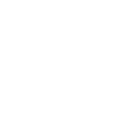TOCEC##### 邏輯學,科學總論,工程
 層級： 課程資料 媒體： 影音

# 11002控制系統一(重新檢測版= 10410ing)

2021-08-07-2030-12-31

This course provides the students with basic knowledge in modeling, analysis and design for linear feedback control systems.  It begins with reviewing some mathematical fundamentals and introducing block diagrams and signal-flow graphs.  Students will then learn how to model mechanical, electrical......

【課程大綱】
Outline of this course
This course provides the students with basic knowledge in modeling, analysis and design for linear feedback control systems.  It begins with reviewing some mathematical fundamentals and introducing block diagrams and signal-flow graphs.  Students will then learn how to model mechanical, electrical, and electromechanical systems as differential equations and transfer functions.  The analysis in this course includes stability of open-loop and closed-loop systems, time responses and frequency responses of low order systems.  The design methods are divided into root-locus techniques and frequency response techniques using Bode plots for designing PID and lead/lag controllers.  Students will also learn how to apply the automatic control theory to real engineering problems via Matlab simulations and Lab assignments.

【先備知識】
Pre-requisite: Course Contents
 1 Introduction 2 Mathematical Foundation 3 Block diagrams and Signal-Flow Graphs 4 Modeling of Dynamic Systems 5 Time-domain Analysis of Control Systems 6 Root Locus Analysis 7 Frequency-Domain Analysis 8 Design of Control Systems

https://ocw.nthu.edu.tw/ocw/index.php?page=course&cid=306&